# Addition Equations Subtraction Equations Multiplication Equations Division Equations

• Slides: 51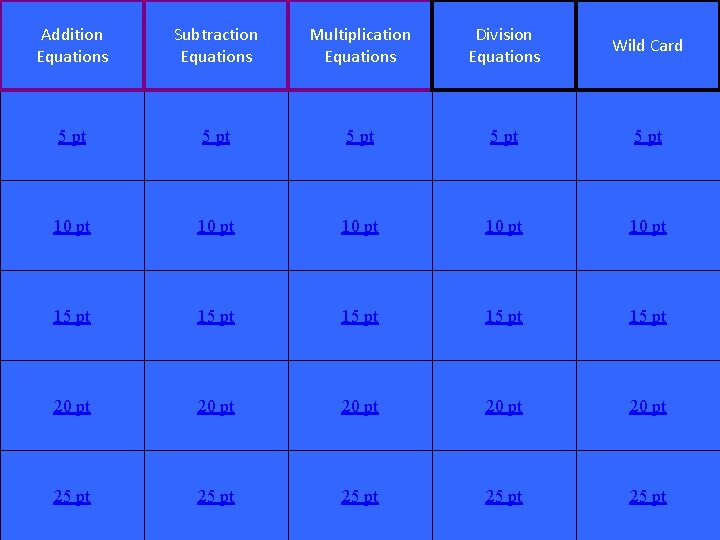Addition Equations Subtraction Equations Multiplication Equations Division Equations Wild Card 5 pt 5 pt 10 pt 10 pt 15 pt 15 pt 20 pt 20 pt 25 pt 25 pt 1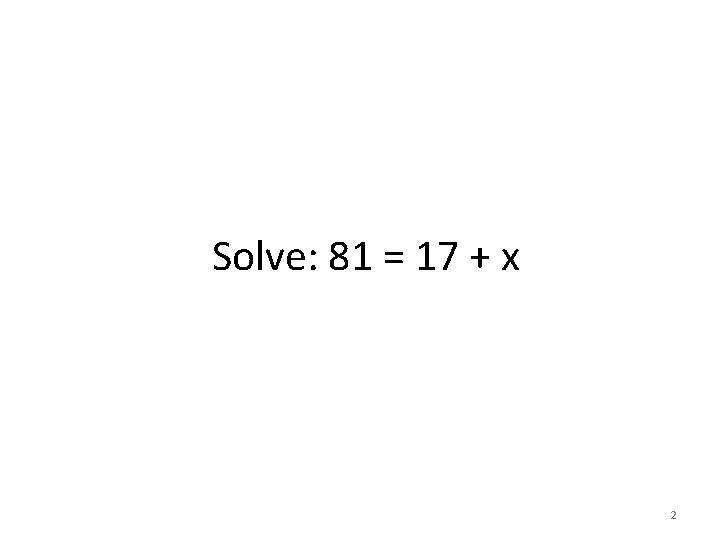Solve: 81 = 17 + x 2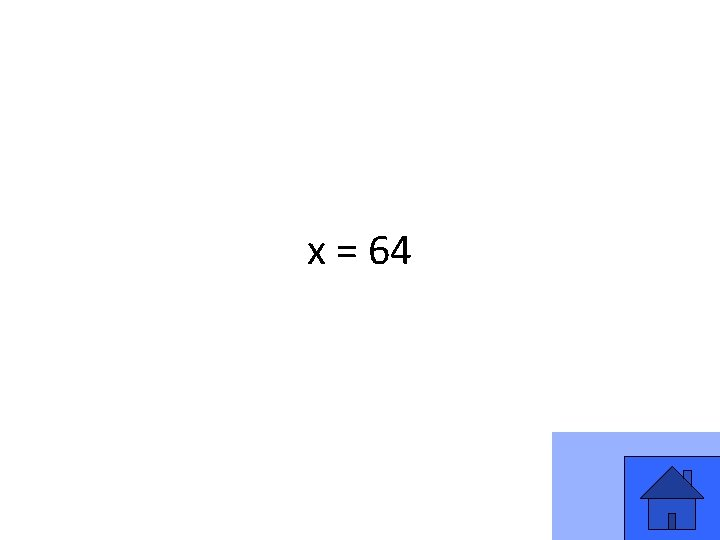x = 64 3Solve: x + 62 = 93 4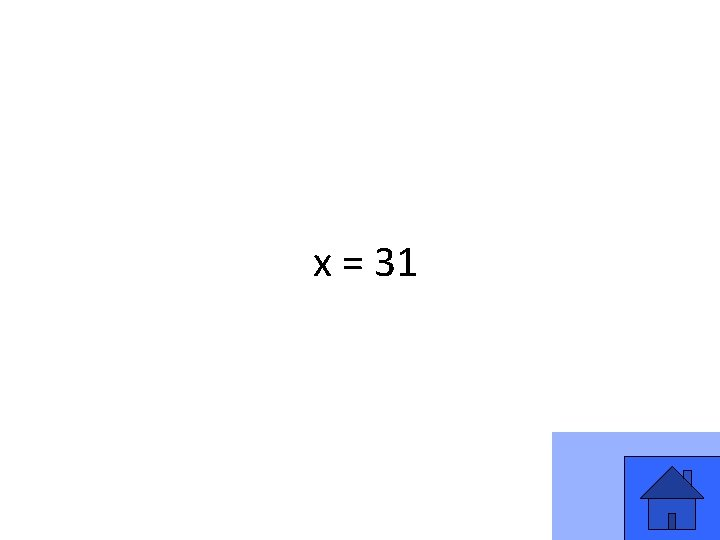x = 31 5Solve: p + 51 = 76 6p = 25 7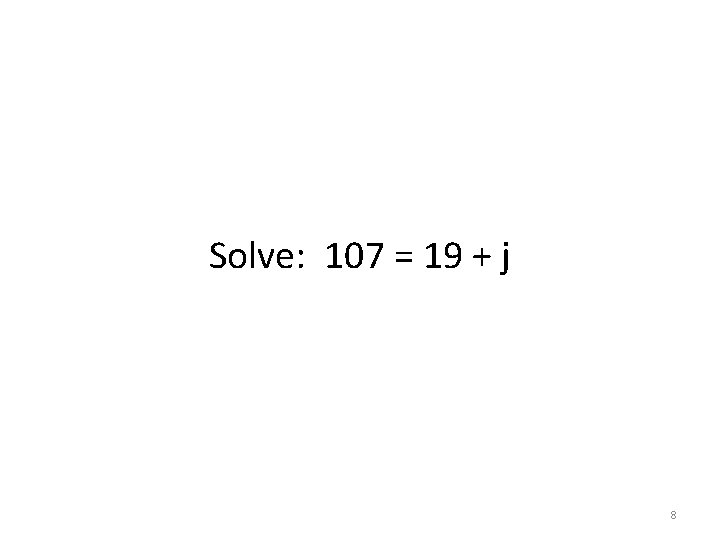Solve: 107 = 19 + j 8j = 88 9Write and solve an equation for this statement: the number of flowers, f, increased by 8 is 27. 10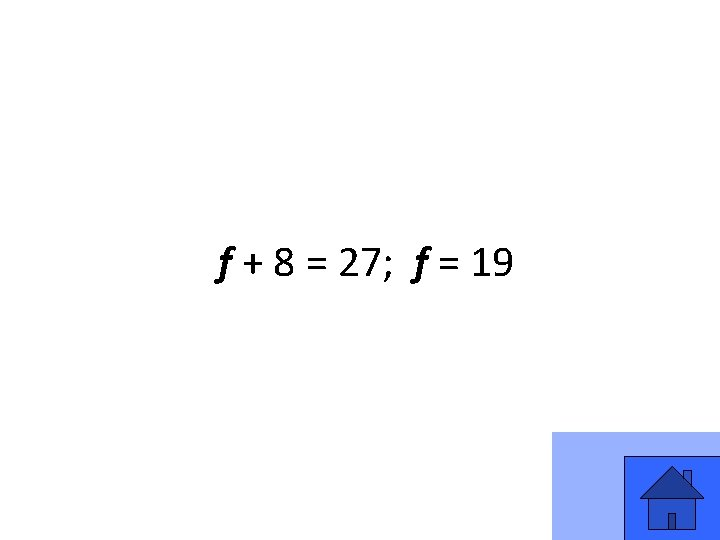f + 8 = 27; f = 19 11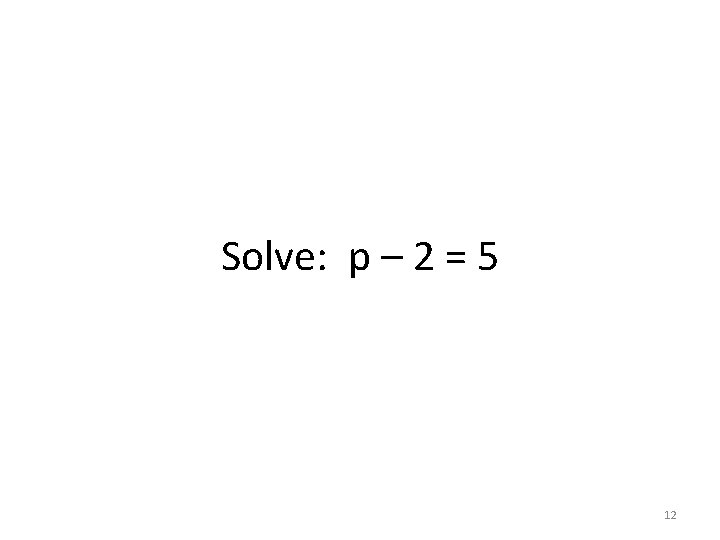Solve: p – 2 = 5 12p=7 13Solve: 40 = x – 11 14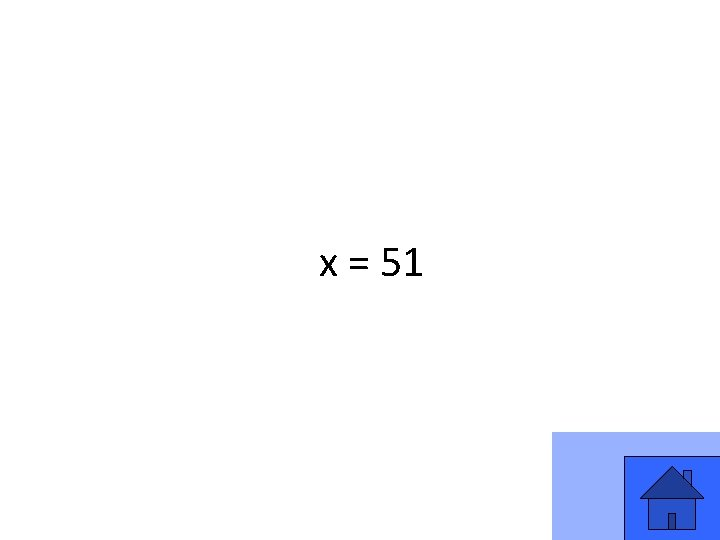x = 51 15Solve: x – 56 = 19 16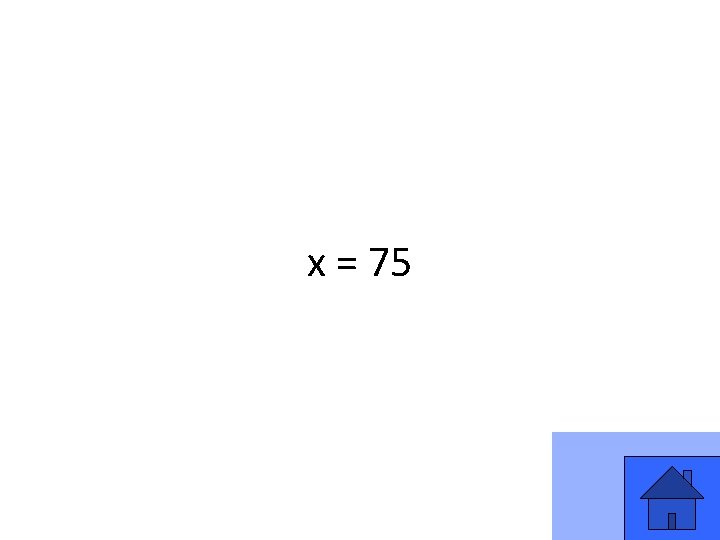x = 75 17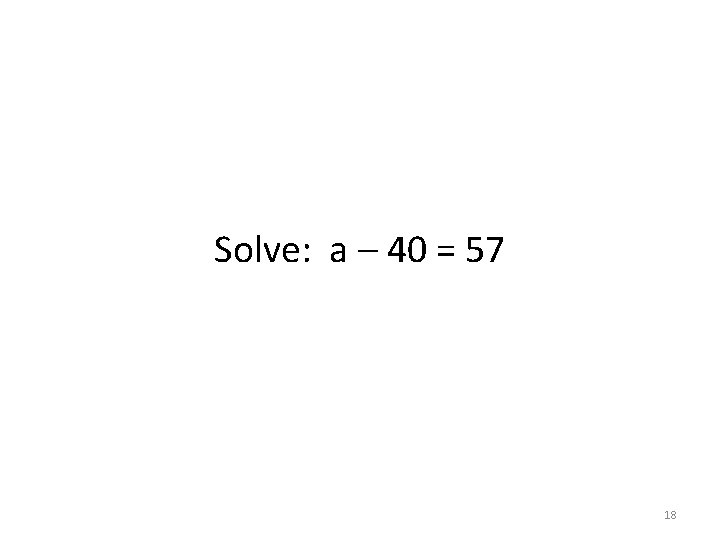Solve: a – 40 = 57 18a = 57 19When 17 is subtracted from a number, the result is 64. Write an equation that can be used to find x. Then, solve for x. 20x – 17 = 64; x = 81 21Solve: 3 x = 12 22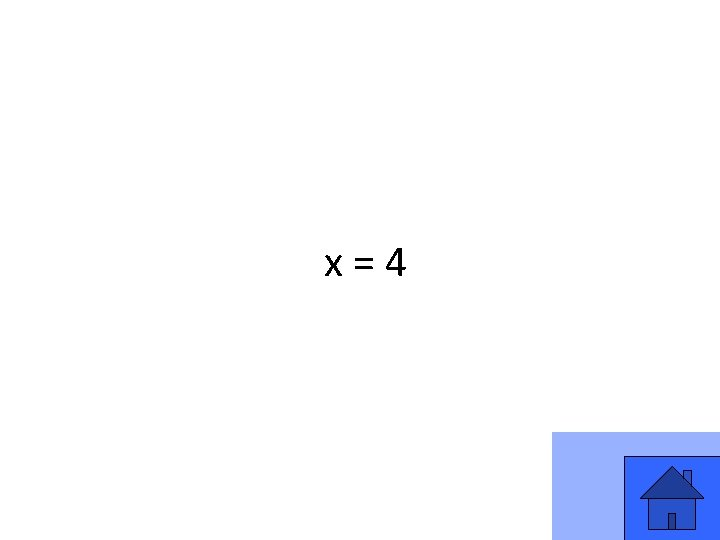x=4 23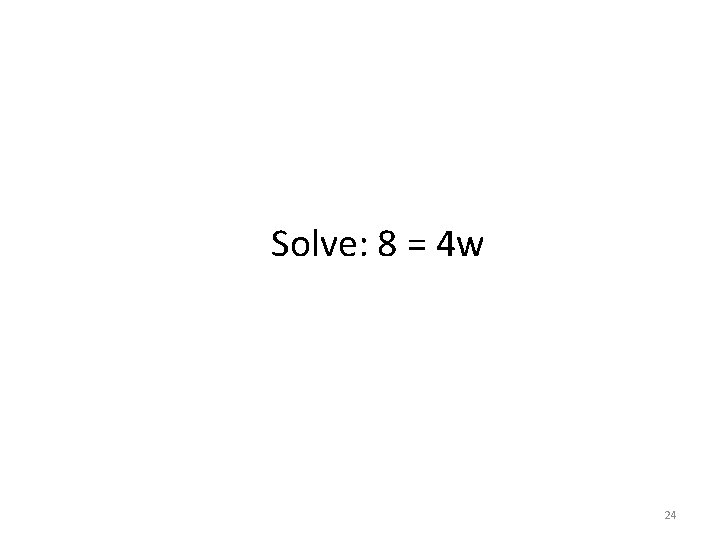Solve: 8 = 4 w 24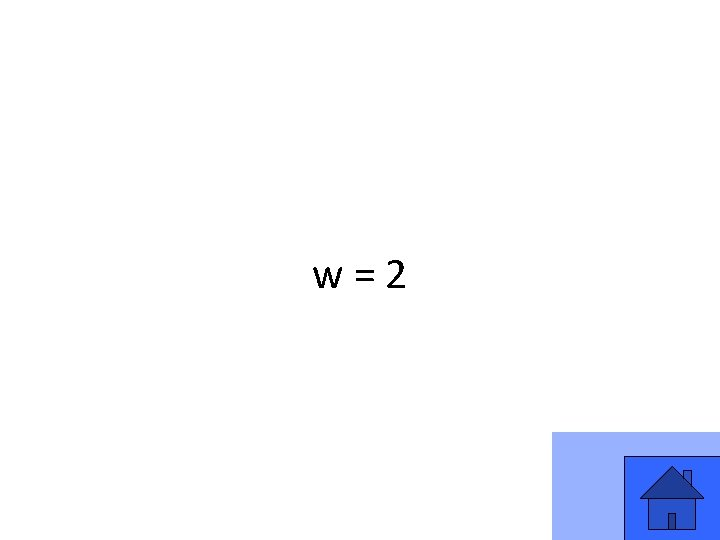w=2 25Solve: 56 = 7 b 26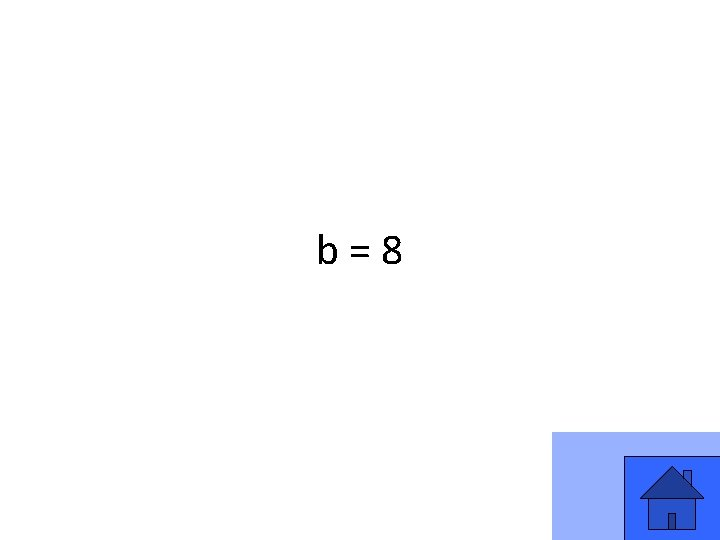b=8 27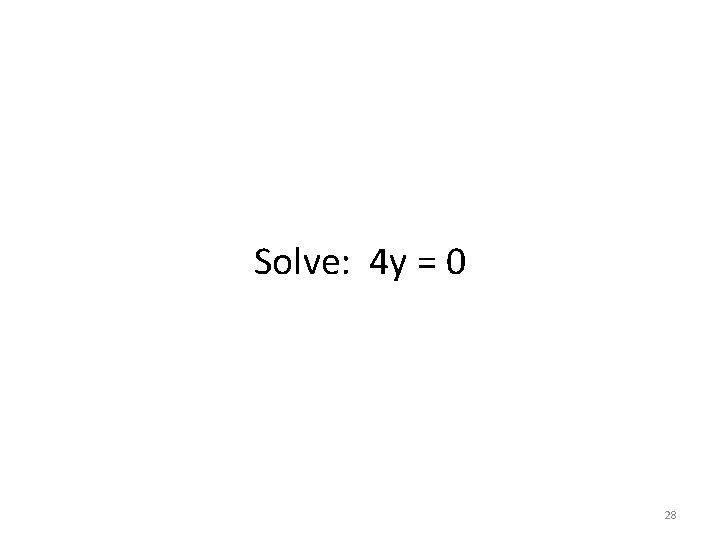Solve: 4 y = 0 28y=0 29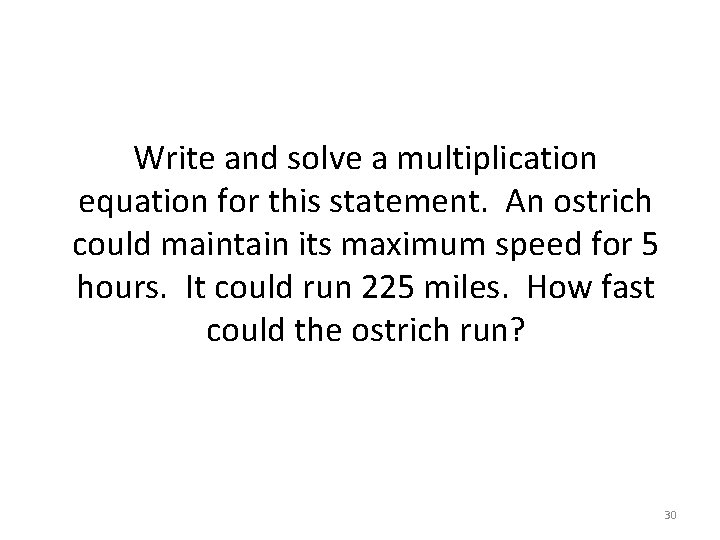Write and solve a multiplication equation for this statement. An ostrich could maintain its maximum speed for 5 hours. It could run 225 miles. How fast could the ostrich run? 305 x = 225; x = 45 miles per hour 31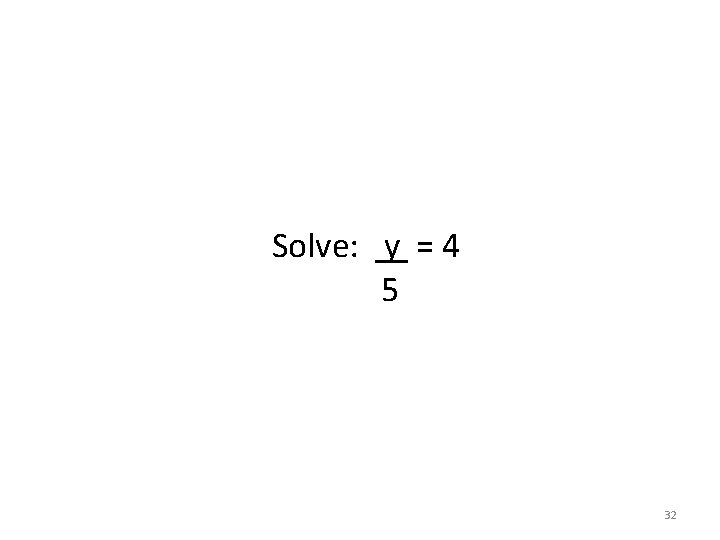Solve: y = 4 5 32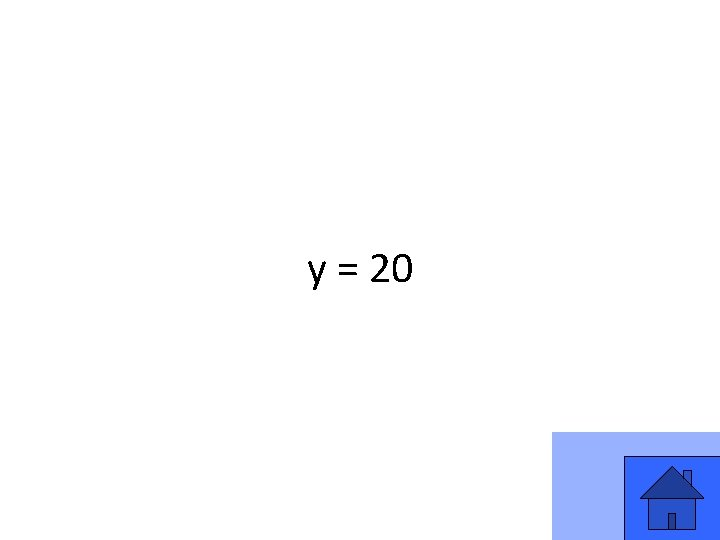y = 20 33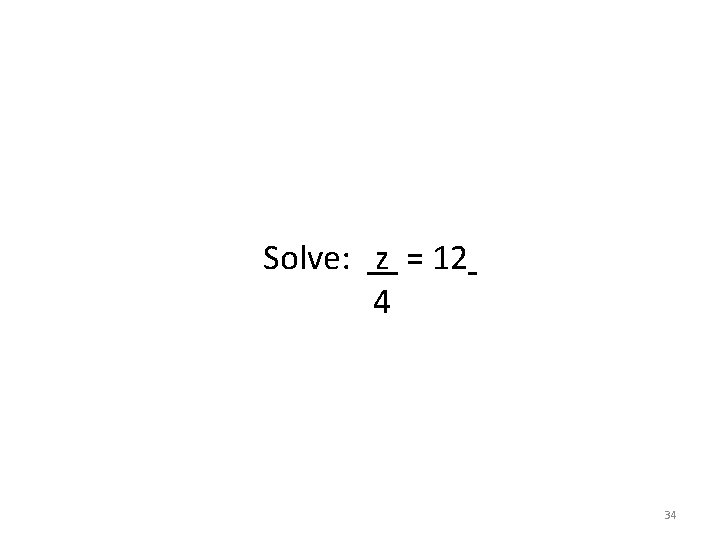Solve: z = 12 4 34z = 48 35Solve: r = 9 9 36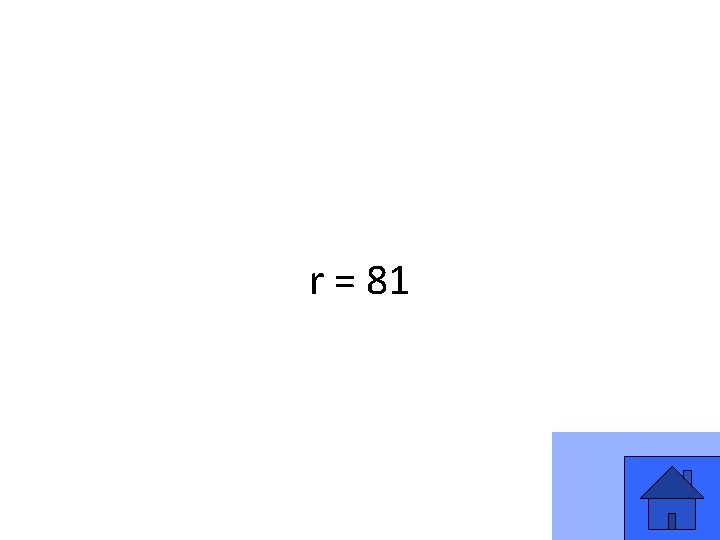r = 81 37Solve: c = 11 15 38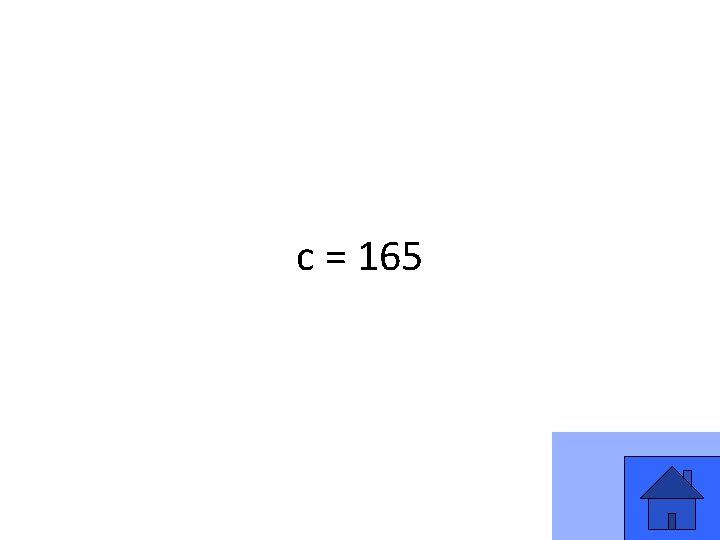c = 165 39A number halved and then halved again is equal to 2. What was the original number? 40The original number is 8. (8 ÷ 2 = 4; 4 ÷ 2 = 2) 41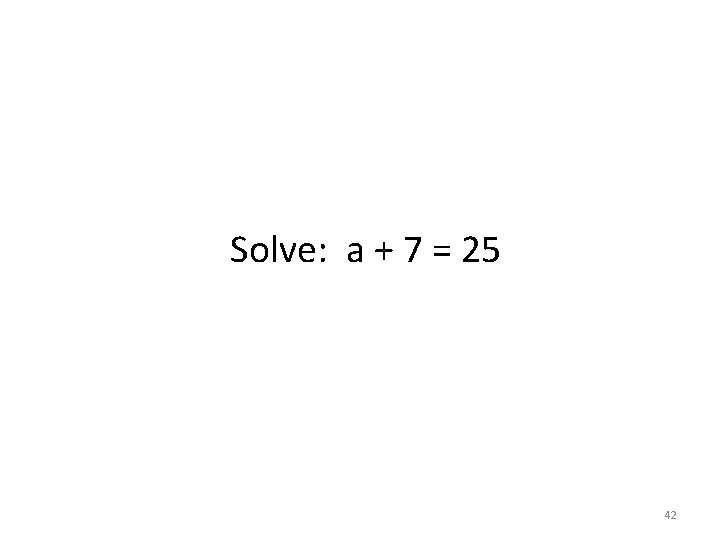Solve: a + 7 = 25 42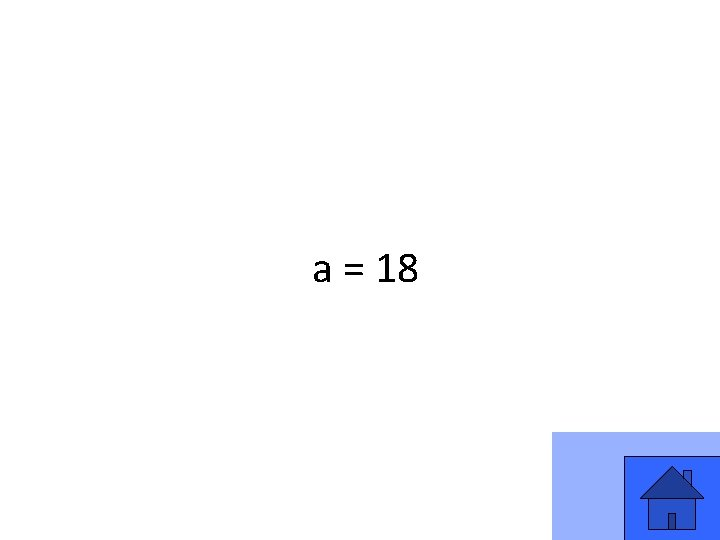a = 18 43Solve: 121 = 11 d 44d = 11 45Solve: 3 = t – 8 46t = 11 47Solve: k = 6 9 48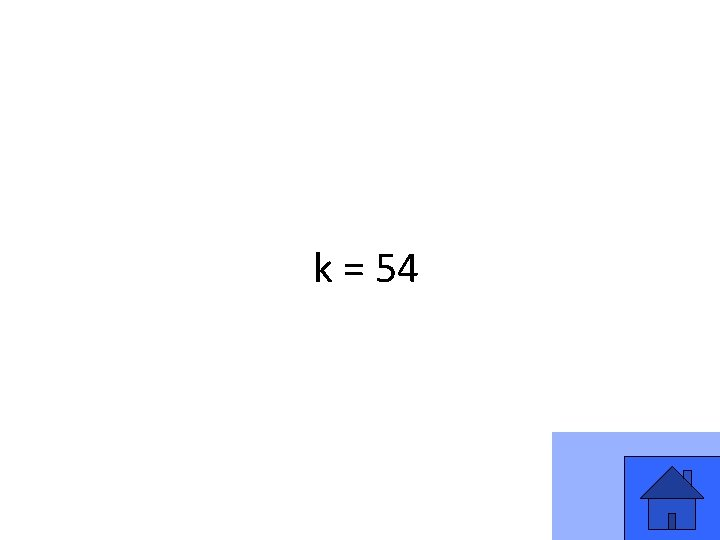k = 54 49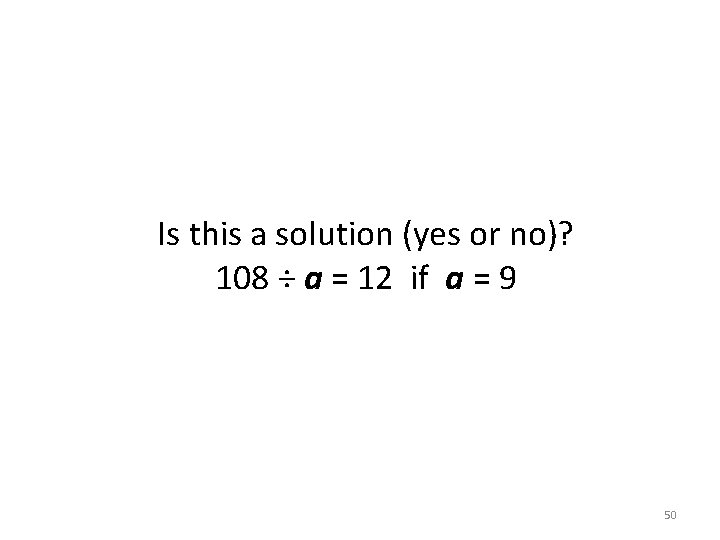Is this a solution (yes or no)? 108 ÷ a = 12 if a = 9 50Yes, it is a solution. 51